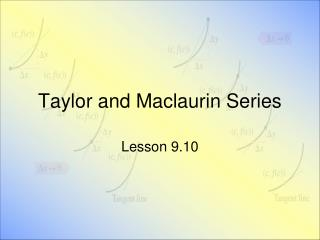DownloadDownload PresentationTaylor and Maclaurin Series

# Taylor and Maclaurin Series

Télécharger la présentation## Taylor and Maclaurin Series

- - - - - - - - - - - - - - - - - - - - - - - - - - - E N D - - - - - - - - - - - - - - - - - - - - - - - - - - -
##### Presentation Transcript

1. Taylor and Maclaurin Series Lesson 9.10

2. Convergent Power Series Form • Consider representing f(x) by a power series • For all x in open interval I • Containing c • Then

3. Taylor Series • If a function f(x) has derivatives of all orders at x = c, then the seriesis called the Taylor series for f(x) at c. • If c = 0, the series is the Maclaurin series for f .

4. Taylor Series • This is an extension of the Taylor polynomials from section 9.7 • We said for f(x) = sin x, Taylor Polynomial of degree 7

5. Guidelines for Finding Taylor Series • Differentiate f(x) several times • Evaluate each derivative at c • Use the sequence to form the Taylor coefficients • Determine the interval of convergence • Within this interval of convergence, determine whether or not the series converges to f(x)

6. Series for Composite Function • What about when f(x) = cos(x2)? • Note the series for cos x • Now substitute x2 in for the x's

7. Binomial Series • Consider the function • This produces the binomial series • We seek a Maclaurin series for this function • Generate the successive derivatives • Determine • Now create the series using the pattern

8. Binomial Series • We note that • Thus Ratio Test tells us radius of convergence R = 1 • Series converges to some function in interval-1 < x < 1

9. Combining Power Series • Consider • We know • So we could multiply and collect like terms

10. Assignment • Lesson 9.10 • Page 685 • 1 – 29 odd